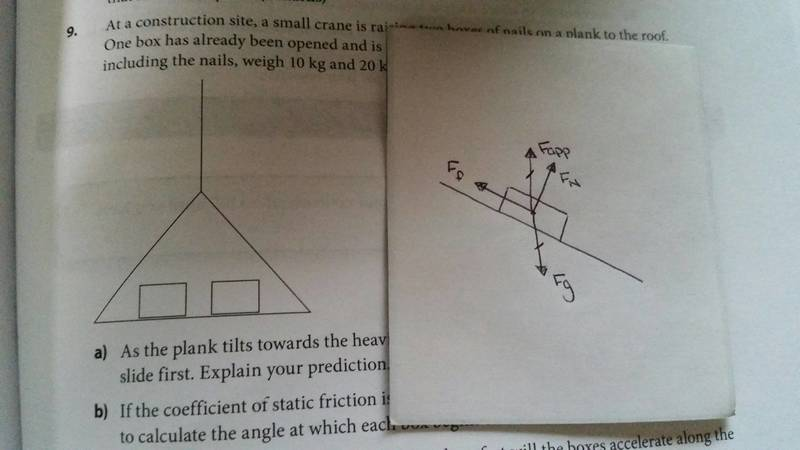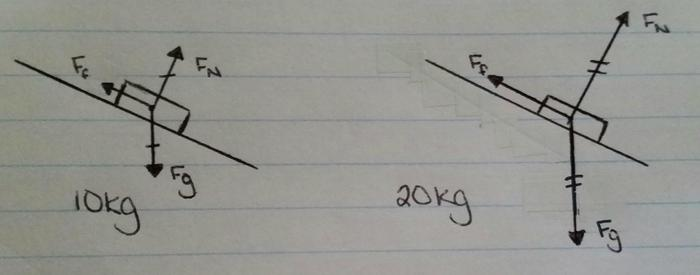# Help Drawing Free Body Diagrams?

• Misiu
See if you can figure it out! You know that the force of gravity must change, so how would that impact the other forces?The force of gravity would change the normal force and the friction force, but the other two forces would stay the same. The force of friction would be longer, and the force of gravity would be shorter.See if you can figure it out! You know that the force of gravity must change, so how would that impact the other forces?The force of gravity would change the normal force and the friction force, but the other two forces would stay the same. The force of friction would be longer, and the force of gravity would be shorter.

## Homework Statement

At a construction site, a small crane is raising two boxes of nails on a plank to the roof. One box has already been opened and is half full, while the other box is new. The boxes, including the nails, weigh 10kg and 20kg, respectively, and are both the same size.

If the coefficient of static friction is 0.4, draw a FBD for each box of nails and use it to calculate the angle at which each box begins to slide.

N/A

## The Attempt at a Solution

I can figure out the calculations/the angle just fine (I believe the answer is 22 degrees), I just struggle with drawing FBD. I'm confused as to how many forces are acting on the boxes. The obvious one is a force of gravity down, and I'm guessing the normal force would be on a slant (perpendicular to the slant of the plank since it's uneven with the weight). There's the force of friction (Is this heading downward or upward?)

I attempted my drawing here:The lines are a bit shaky since it was just an attempt, but am I on the right track or did I forget something? Thanks in advance! :D

Last edited by a moderator:
Misiu said:
The obvious one is a force of gravity down, and I'm guessing the normal force would be on a slant (perpendicular to the slant of the plank since it's uneven with the weight).
All good. Realize that "normal" means perpendicular to the surface.

Misiu said:
There's the force of friction (Is this heading downward or upward?)
Since the friction is helping to prevent slipping, it had better be acting up the incline, just as you drew it.

What's the Fapp that I see in the diagram? There's no such force.

Doc Al said:
What's the Fapp that I see in the diagram? There's no such force.

The question says that the crane is raising the boxes to the roof. Is Fapp the incorrect force and should it be something else instead?

Misiu said:
The question says that the crane is raising the boxes to the roof. Is Fapp the incorrect force and should it be something else instead?
The crane does not act directly on the boxes. The only thing touching the boxes is the plank, so the only forces acting on the boxes (besides gravity) are exerted by the plank. The normal force and the friction force already captures those forces.

Doc Al said:
The crane does not act directly on the boxes. The only thing touching the boxes is the plank, so the only forces acting on the boxes (besides gravity) are exerted by the plank. The normal force and the friction force already captures those forces.

Alright, thanks!

The question also says to draw a FBD for each of the boxes. Does the mass impact any of the forces or would the two have identical FBD?

Misiu said:
Does the mass impact any of the forces or would the two have identical FBD?
Yes and yes. ;) (Note that the forces in your diagram are not necessarily drawn to scale.)

Doc Al said:
Yes and yes. ;) (Note that the forces in your diagram are not necessarily drawn to scale.)

Now I'm confused, you answered yes to the boxes having different and identical FBD.I was going to ask, would the heavier box (20kg) have a longer friction line than the 10kg box? I think the other two forces would stay the same length for both boxes (or maybe gravity would be a longer line as well for the heavier box, not sure about the normal force), but I could be wrong about that.

Thanks again for your continued help! :)

Misiu said:
Now I'm confused, you answered yes to the boxes having different and identical FBD.
I was being a bit sneaky, to get you thinking. You asked if the forces would change. Sure. The magnitudes of the forces will change, but your free body diagram will show the same three generic forces--it doesn't reflect the magnitudes.

Misiu said:
I was going to ask, would the heavier box (20kg) have a longer friction line than the 10kg box? I think the other two forces would stay the same length for both boxes (or maybe gravity would be a longer line as well for the heavier box, not sure about the normal force), but I could be wrong about that.
See if you can figure it out! You know that the force of gravity must change, so how would that impact the other forces?

Doc Al said:
See if you can figure it out! You know that the force of gravity must change, so how would that impact the other forces?So, like this? Making sure that normal force equaled the force of gravity for both boxes, with the heavier box having a longer force of gravity than the lighter one. The force of friction would also be longer, I think.

Misiu said:
Making sure that normal force equaled the force of gravity for both boxes
You think those forces are equal? Why?

Misiu said:
with the heavier box having a longer force of gravity than the lighter one.
Good.

Misiu said:
The force of friction would also be longer, I think.
Yep.

Doc Al said:
You think those forces are equal? Why?

I thought the normal force always equaled the force of gravity. Is that not the case here? :(

Misiu said:
I thought the normal force always equaled the force of gravity. Is that not the case here?
That would be true if the plank were horizontal. But it's not. (What must be true is that the sum of the forces perpendicular to the surface add to zero.)

Doc Al said:
That would be true if the plank were horizontal. But it's not. (What must be true is that the sum of the forces perpendicular to the surface add to zero.)

Hmm.. in that case, the normal force would be shorter for both boxes than the force of gravity? Not understanding the part in brackets. It would equal the force of friction?

Misiu said:
Hmm.. in that case, the normal force would be shorter for both boxes than the force of gravity?
That's right. The normal force equals the component of gravity perpendicular to the surface.